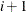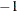### Output Data Sets

The procedure determines the flow that should pass through each arc as well as the value assigned to each nonarc variable. The goal is that the minimum flow bounds, capacities, lower and upper value bounds, and side constraints are not violated. This goal is reached when total cost incurred by such a flow pattern and value assignment is feasible and optimal. The solution found must also conserve flow at each node.

The ARCOUT= data set contains a solution obtained when performing optimization that does not consider any constraints. The NODEOUT= data set contains nodal dual variable information for this type of solution. You can choose to have PROC NETFLOW create the ARCOUT= data set and the NODEOUT= data set and save the optimum of the network or the nodal dual variable values before any optimization that considers the side constraints is performed.

If there are side constraints, the CONOUT= data set can be produced and contains a solution obtained after performing optimization that considers constraints. The DUALOUT= data set contains dual variable information for nodes and side constraints from the solution obtained after optimization that considers the constraints. The CONOUT= data set and DUALOUT= data set can be used to save the constrained optimal solution.

#### ARCOUT= and CONOUT= Data Sets

The ARCOUT= and CONOUT= data sets contain the same variables. Furthermore, the variables in the output data sets depend on whether or not the problem has a network component.

If the problem has a network component, the variables and their possible values in an observation are as follows:

 `_FROM_` a tail node of an arc. This is a missing value if an observation has information about a nonarc variable. `_TO_` a head node of an arc. This is a missing value if an observation has information about a nonarc variable. `_COST_` the cost of an arc or the objective function coefficient of a nonarc variable `_CAPAC_` the capacity of an arc or upper value bound of a nonarc variable `_LO_` the lower flow bound of an arc or lower value bound of a nonarc variable `_NAME_` a name of an arc or nonarc variable `_SUPPLY_` the supply of the tail node of the arc in the observation. This is a missing value if an observation has information about a nonarc variable. `_DEMAND_` the demand of the head node of the arc in the observation. This is a missing value if an observation has information about a nonarc variable. `_FLOW_` the flow through the arc or value of the nonarc variable `_FCOST_` flow cost, the product of `_COST_` and `_FLOW_` `_RCOST_` the reduced cost of the arc or nonarc variable `_ANUMB_` the number of the arc (positive) or nonarc variable (nonpositive); used for warm starting PROC NETFLOW `_TNUMB_` the number of the tail node in the network basis spanning tree; used for warm starting PROC NETFLOW `_STATUS_` the status of the arc or nonarc variable

If the problem does not have a network component, the variables and their possible values in an observation are as follows:

 `_OBJFN_` the objective function coefficient of a variable `_UPPERBD` the upper value bound of a variable `_LOWERBD` the lower value bound of a variable `_NAME_` the name of a variable `_VALUE_` the value of the variable `_FCOST_` objective function value for that variable; the product of `_OBJFN_` and `_VALUE_`

The variables present in the ARCDATA= data set are present in an ARCOUT= data set or a CONOUT= data set. For example, if there is a variable called `tail` in the ARCDATA= data set and you specified the SAS variable list

```  from tail;
```

then `tail` is a variable in the ARCOUT= and CONOUT= data sets instead of `_FROM_`. Any ID list variables also appear in the ARCOUT= and CONOUT= data sets.

#### NODEOUT= and DUALOUT= Data Sets

There are two types of observations in the NODEOUT= and DUALOUT= data sets. One type of observation contains information about a node. These are called type N observations. There is one such observation of this type for each node. The `_NODE_` variable has a name of a node, and the `_CON_` variable values in these observations are missing values.

The other type of observation contains information about constraints. These are called the type C observations. There is one such observation for each constraint. The `_CON_` variable has a name of a constraint, and the `_NODE_` variable values in these observations are missing values.

Many of the variables in the NODEOUT= and DUALOUT= data sets contain information used to warm start PROC NETFLOW. The variables `_NODE_`, `_SD_`, `_DUAL_`, `_VALUE_`, `_RHS_`, `_TYPE_`, and `_CON_` contain information that might be of interest to you.

The NODEOUT= and DUALOUT= data sets look similar, as the same variables are in both. These variables and their values in an observation of each type are

 `_NODE_` Type N: the node name Type C: a missing value `_SD_` Type N: the supply (positive) or demand (negative) of the node Type C: a missing value `_DUAL_` Type N: the dual variable value of the node in `_NODE_` Type C: the dual variable value of the constraint named in `_CON_` `_NNUMB_` Type N: the number of the node named in `_NODE_` Type C: the number of the constraint named in `_CON_` `_PRED_` Type N: the predecessor in the network basis spanning tree of the node named in `_NODE_` Type C: the number of the node toward which the arc with number in `_ARCID_` is directed, or the constraint number associated with the slack, surplus, or artificial variable basic in this row `_TRAV_` Type N: the traversal thread label of the node named in `_NODE_` Type C: a missing value `_SCESS_` Type N: the number of successors (including itself) in the network basis spanning tree of the node named in `_NODE_` Type C: a missing value `_ARCID_` Type N: if `_ARCID_` is nonnegative, `_ARCID_` is the number of the network basis spanning tree arc directed from the node with number `_PRED_` to the node named in `_NODE_`. If `_ARCID_` is negative, minus `_ARCID_` is the number of the network basis spanning tree arc directed from the node named in `_NODE_` to the node with number `_PRED_`. Type C: if `_ARCID_` is positive, `_ARCID_` is the number of the arc basic in a constraint row. If nonpositive, minus `_ARCID_` is the number of the nonarc variable basic in a constraint row. `_FLOW_` Type N: the flow minus the lower flow bound of the arc `_ARCID_` Type C: the flow minus lower flow bound of the arc `_ARCID_` or value lower bound of the nonarc variable value minus `_ARCID_` `_FBQ_` Type N: If `_FBQ_` is positive, then `_FBQ_` is the subscript in arc length arrays of the first arc directed toward the node named in `_NODE_`. PROC NETFLOW’s arc length arrays are sorted so that data of arcs directed toward the same head node are together. If `_FBQ_` is negative, no arcs are directed toward the node named in `_NODE_`. Arcs directed toward nodehave subscripts in the arc length arrays between observations FBQ() and (FBQ()), inclusive. Type C: a missing value `_VALUE_` Type N: a missing value Type C: the lhs value (the sum of the products of coefficient and flows or values) of the constraint named in `_CON_` `_RHS_` Type N: a missing value Type C: the rhs value of the constraint named in `_CON_` `_TYPE_` Type N: a missing value Type C: the type of the constraint named in `_CON_` `_CON_` Type N: a missing value Type C: the name of the constraint

If specified in variable lists, the variables in the input data sets are used instead of some of the previous variables. These variables are specified in the NODE, SUPDEM, RHS, TYPE, and ROW (if there is only one variable in the ROW list) lists and are used instead of `_NODE_`, `_SD_`, `_RHS_`, `_TYPE_`, and `_CON_`, respectively.

#### MPSOUT= Data Set

The MPSOUT= data set contains problem data converted from a PROC NETFLOW format into an MPS-format SAS data set. The six fields, `FIELD1` to `FIELD6`, in the MPSOUT= data set correspond to the six columns in MPS standard. For more information about the MPS-format SAS data set, see Chapter 15: The MPS-Format SAS Data Set in SAS/OR 12.3 User's Guide: Mathematical Programming.